# Plotting

``library(faux)``

## Plotting Designs

The functions `check_design()` and `sim_design()` will automatically create plots of your design (unless you set `plot = FALSE`) so you can check you set it up correctly. You can also use the `plot_design()` function to plot a saved design list.

### One-factor designs

``````p1 <- check_design(plot = FALSE) %>% plot_design()
p2 <- check_design(2, plot = FALSE) %>% plot_design()
p3 <- check_design(3, plot = FALSE) %>% plot_design()
p4 <- check_design(4, plot = FALSE) %>% plot_design()
p5 <- check_design(5, plot = FALSE) %>% plot_design()
p6 <- check_design(6, plot = FALSE) %>% plot_design()

cowplot::plot_grid(p1, p2, p3, p4, p5, p6, nrow = 2)``````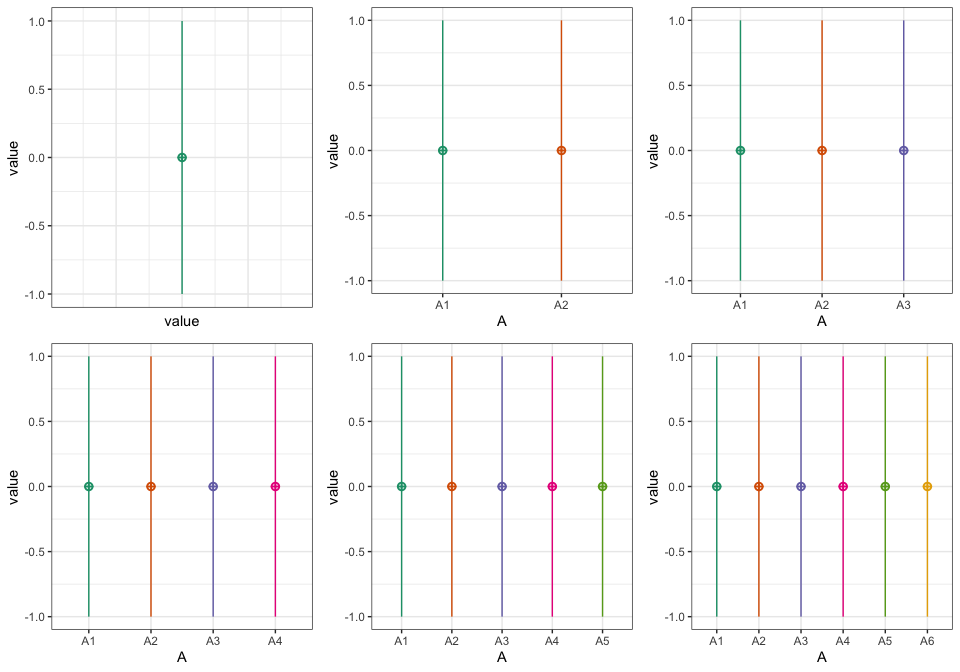### Two-factor designs

``````p1 <- check_design(c(2,2), mu = 1:4, plot = FALSE) %>% plot_design()
p2 <- check_design(c(2,3), mu = 1:6, plot = FALSE) %>% plot_design()
p3 <- check_design(c(2,4), mu = 1:8, plot = FALSE) %>% plot_design()
p4 <- check_design(c(3,2), mu = 1:6, plot = FALSE) %>% plot_design()
p5 <- check_design(c(3,3), mu = 1:9, plot = FALSE) %>% plot_design()
p6 <- check_design(c(3,4), mu = 1:12, plot = FALSE) %>% plot_design()

cowplot::plot_grid(p1, p2, p3, p4, p5, p6, nrow = 3)``````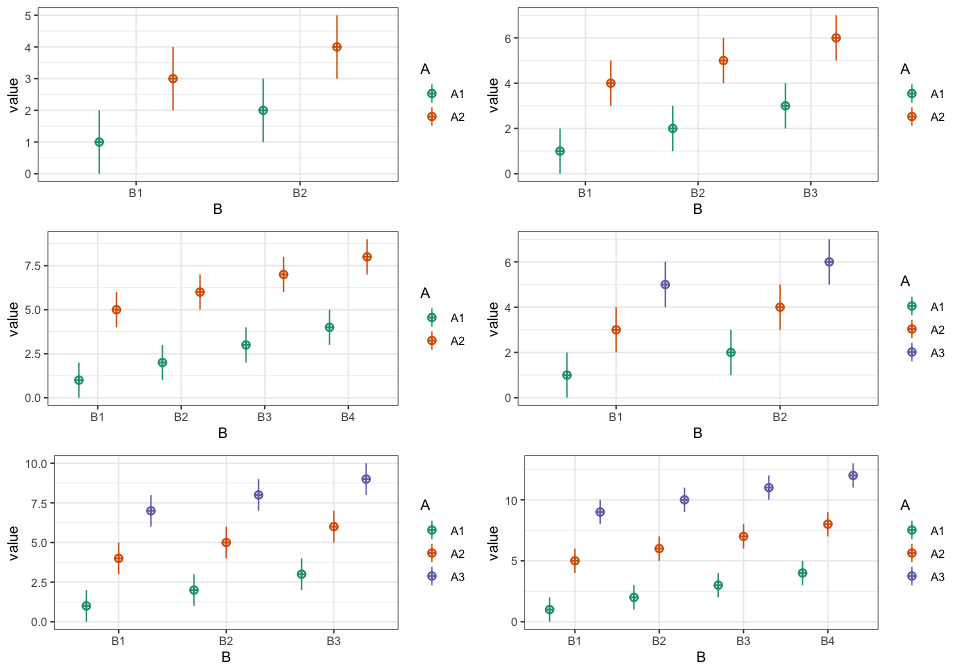### Three-factor designs

``check_design(c(2,2,2), mu = 1:2^3)``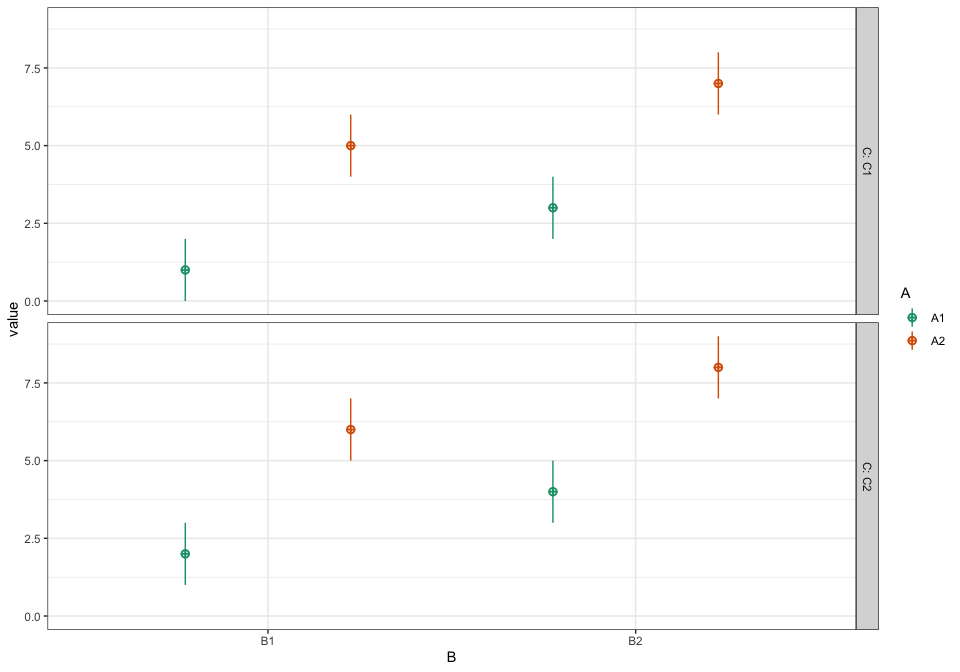### Four-factor designs

``check_design(c(2,2,2,2), mu = 1:2^4)``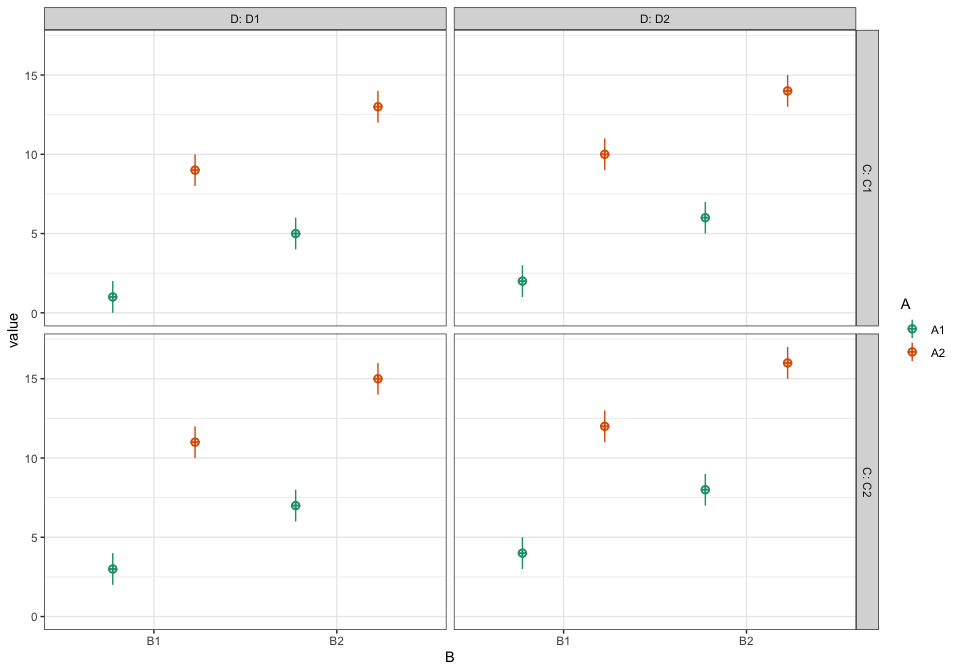### Five-factor designs

``check_design(c(2,2,2,2,2), mu = 1:(2^5))``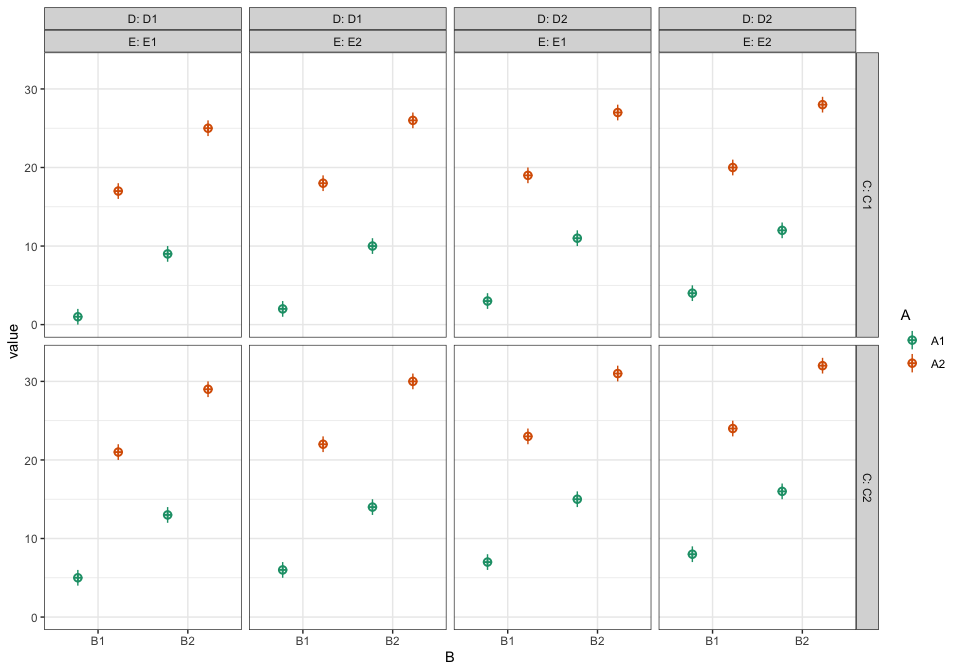### Six-factor designs

``check_design(c(2,2,2,2,2,2), mu = 1:(2^6))``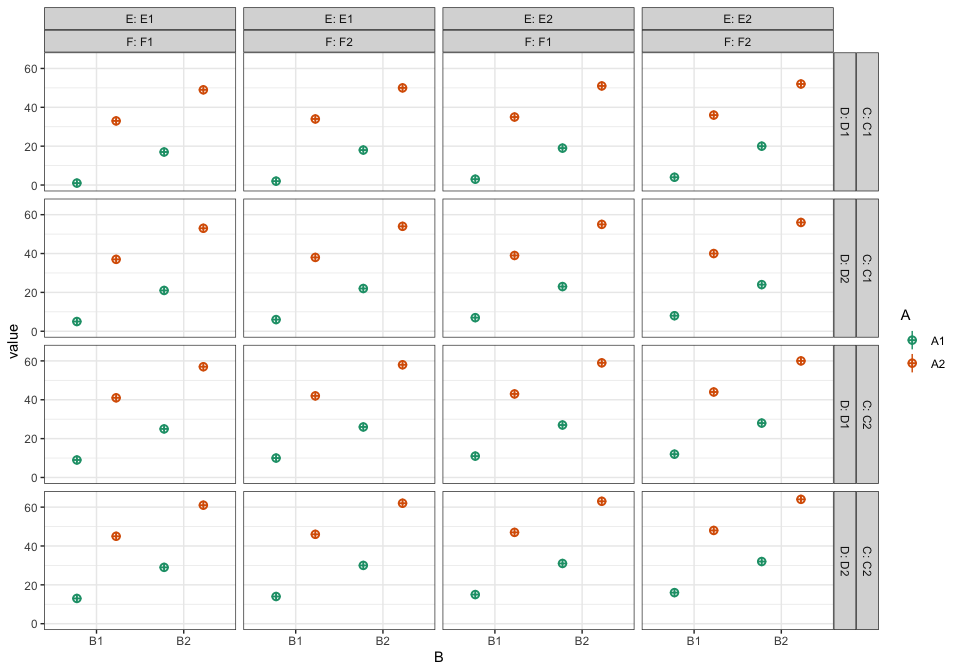## Plotting Data

You can plot data created with faux using `plot_design()`, too. It will return a ggplot with a violin-boxplot by default.

### One-factor data

``````data <- sim_design(2, 2, n = 20, mu = 1:4, plot = FALSE)
plot_design(data)``````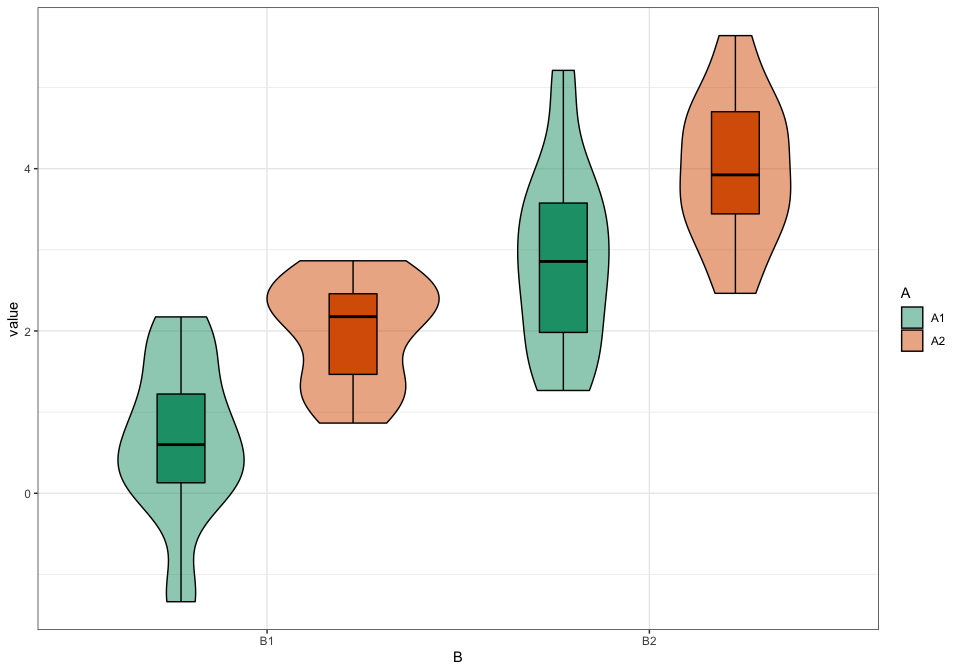``plot_design(data, geoms = "violin")``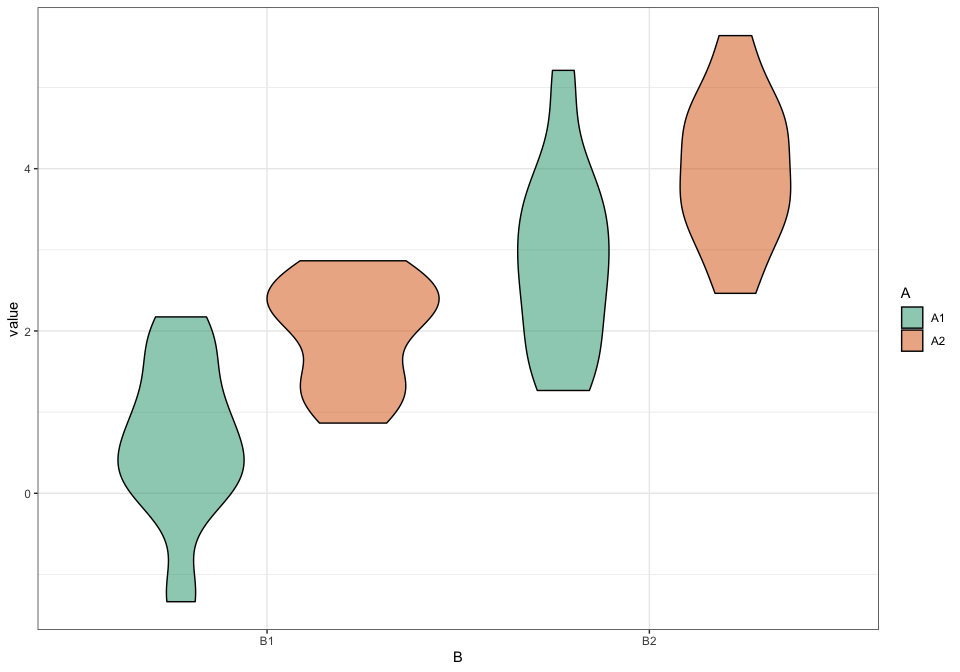``plot_design(data, geoms = "box")``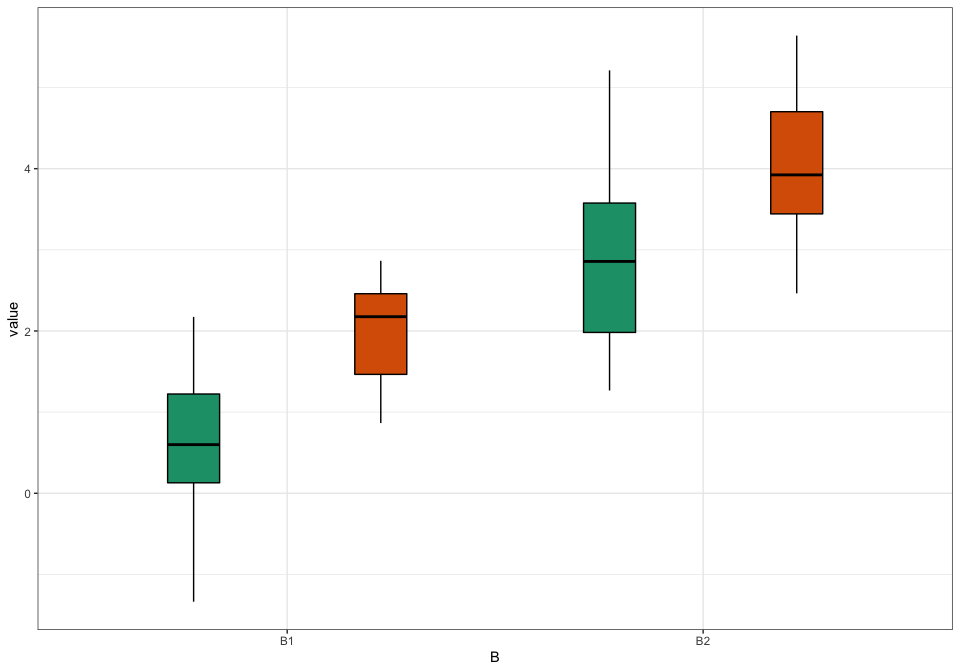``plot_design(data, geoms = "pointrangeSD")``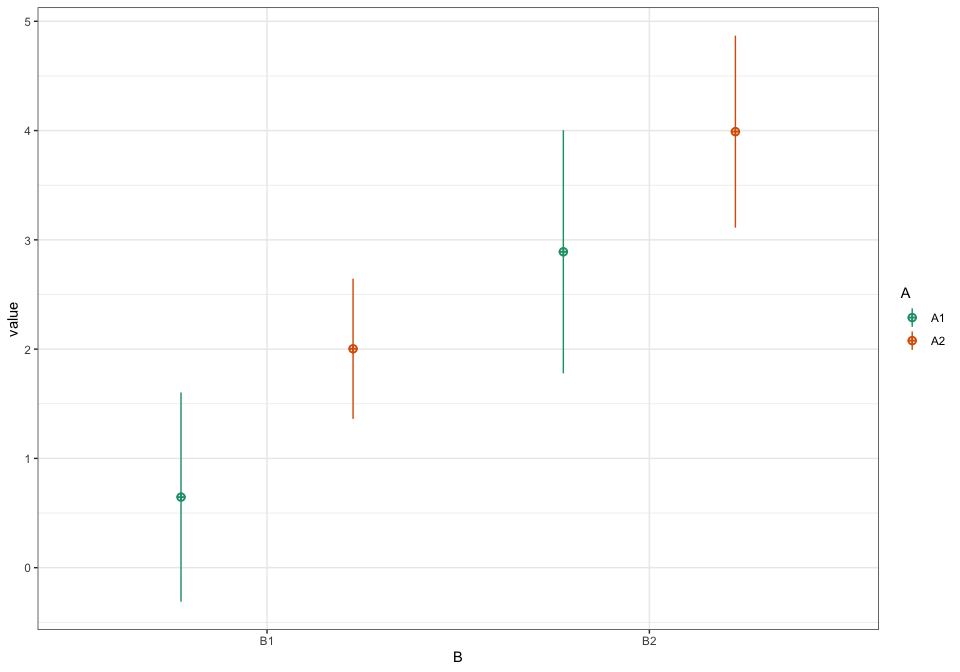``plot_design(data, geoms = c("violin", "pointrangeSE"))``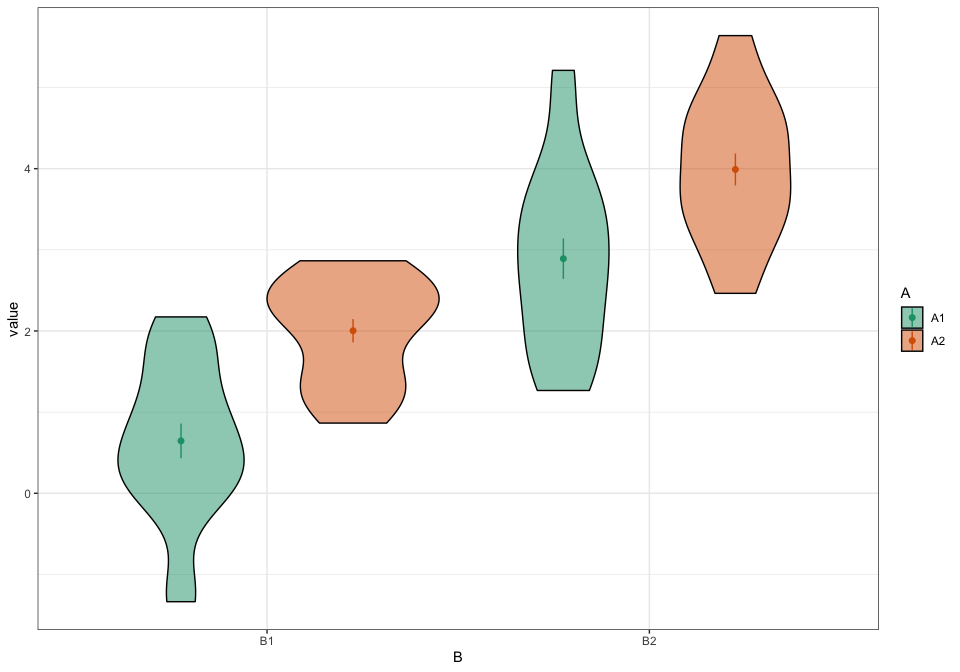``plot_design(data, geoms = c("violin", "jitter"))``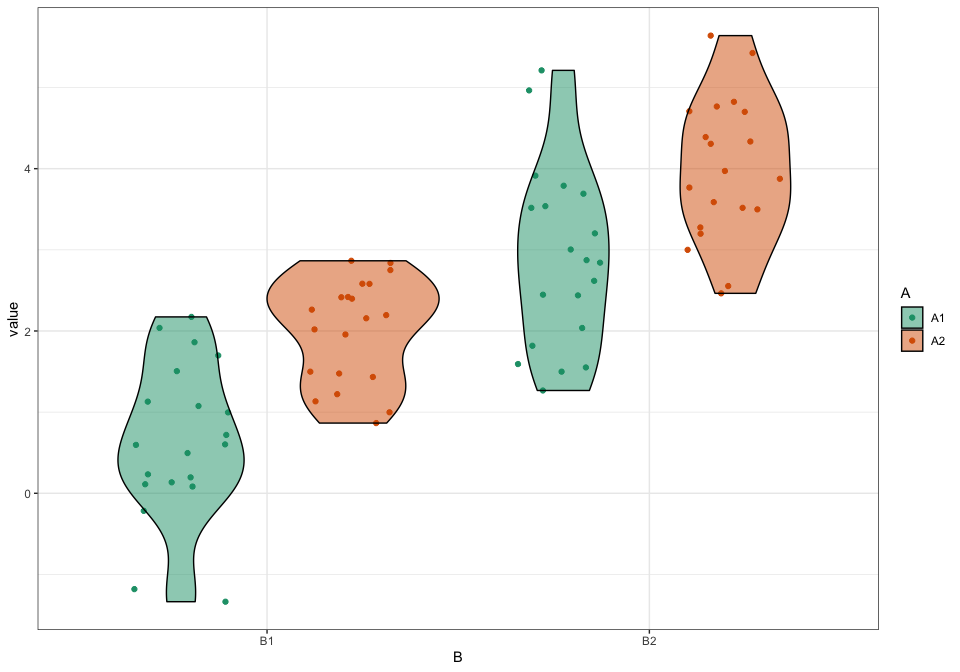### Two-factor data

``````data <- sim_design(2, 2, mu = 1:4, plot = FALSE)
plot_design(data)``````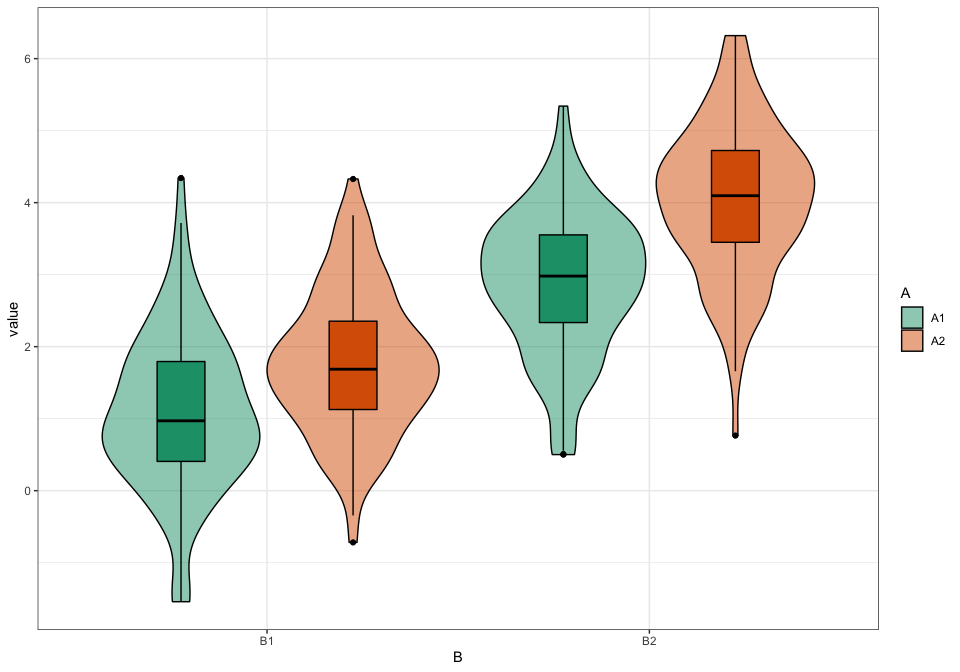### Change order of factors

``````data <- sim_design(c(2,2,2), n = 50, mu = 1:8, sd = 16, plot = FALSE)
abc <- plot_design(data, "A", "B", "C", geoms = "violin")
acb <- plot_design(data, "A", "C", "B", geoms = "violin")
bac <- plot_design(data, "B", "A", "C", geoms = "violin")
bca <- plot_design(data, "B", "C", "A", geoms = "violin")
cab <- plot_design(data, "C", "A", "B", geoms = "violin")
cba <- plot_design(data, "C", "B", "A", geoms = "violin")

cowplot::plot_grid(abc, acb, bac, bca, cab, cba, nrow = 3,
labels = c("abc", "acb", "bac", "bca", "cab", "cba"))``````